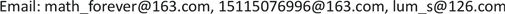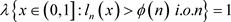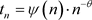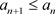﻿ α-Lu¨ roth展式若干度量性质 Some Metric Properties in α-Lu¨ roth Expansions

Vol. 09  No. 01 ( 2020 ), Article ID: 33743 , 6 pages
10.12677/AAM.2020.91002

Some Metric Properties in α-Lüroth Expansions

Bixuan Li, Sha Lan, Luming Shen

Information Science and Technology College of Hunan Agricultural University, Changsha HunanReceived: Dec. 9th, 2019; accepted: Dec. 26th, 2019; published: Jan. 2nd, 2020ABSTRACT

For the α-Lüroth expansion, some metric properties, such as “0-1” law, iterated logarithm law of the digits are studied in this paper. As the extension of alternating-Lüroth expansion, the conclusions in this paper include those of alternating-Lüroth case.

Keywords:α-Lüroth Expansion, “0-1” Law, Iterated Logarithm Lawα-Lüroth展式若干度量性质1. 引言

${L}_{\alpha }\left(x\right):=\left\{\begin{array}{l}\frac{{t}_{n}-x}{{a}_{n}},\text{\hspace{0.17em}}\text{\hspace{0.17em}}x\in {A}_{n},n\in N\\ 0,\text{\hspace{0.17em}}\text{\hspace{0.17em}}\text{\hspace{0.17em}}\text{\hspace{0.17em}}\text{\hspace{0.17em}}\text{\hspace{0.17em}}\text{\hspace{0.17em}}\text{\hspace{0.17em}}\text{\hspace{0.17em}}\text{\hspace{0.17em}}\text{\hspace{0.17em}}\text{\hspace{0.17em}}x=0\end{array}$

$x={t}_{{l}_{1}\left(x\right)}+\underset{j=2}{\overset{\infty }{\sum }}{\left(-1\right)}^{j-1}\left(\underset{1\le i\le j}{\prod }{a}_{{l}_{1}\left(x\right)}\right){t}_{{l}_{j}\left(x\right)}$

1) 如果 $\underset{n=1}{\overset{\infty }{\sum }}\frac{\text{1}}{\varphi \left(n\right)}$ 发散，则 $\lambda \left(A\right)=0$,即2) 如果 $\underset{n=1}{\overset{\infty }{\sum }}\frac{\text{1}}{\varphi \left(n\right)}$ 收敛，则 $\lambda \left\{x\in \left(0,1\right]:{l}_{n}\left(x\right)>\varphi \left(n\right)\text{ }\text{\hspace{0.17em}}i.o.n\right\}=\text{0}$

${L}_{n}\left(x\right)=\mathrm{max}\left\{{l}_{1}\left(x\right),\cdots ,{l}_{n}\left(x\right)\right\}$

$\underset{n\to \infty }{\mathrm{lim}\mathrm{sup}}\frac{\mathrm{log}{l}_{n}\left(x\right)-\mathrm{log}n}{\mathrm{log}\mathrm{log}n}=1$

$\underset{n\to \infty }{\mathrm{lim}\mathrm{sup}}\frac{\mathrm{log}{L}_{n}\left(x\right)-\mathrm{log}n}{\mathrm{log}\mathrm{log}n}=\text{1}$

$\underset{n\to \infty }{\mathrm{lim}\mathrm{inf}}\frac{\mathrm{log}{l}_{n}\left(x\right)-\mathrm{log}n}{\mathrm{log}\mathrm{log}n}=-\infty$

$\underset{n\to \infty }{\mathrm{lim}\mathrm{inf}}\frac{\mathrm{log}{L}_{n}\left(x\right)-\mathrm{log}n}{\mathrm{log}\mathrm{log}n}=0$

2. 准备工作

$I\left({l}_{1},{l}_{2},\cdots ,{l}_{k}\right):=\left\{x\in \left[0,1\right):{l}_{i}\left(x\right)={l}_{i}\text{\hspace{0.17em}}\text{for}\text{\hspace{0.17em}}1\le i\le k\right\}$

1) 若对于 $\rho >1$，有 $\underset{n\to \infty }{\mathrm{lim}}\frac{{t}_{n}}{{t}_{n+1}}=\rho$ ；则 $\alpha$ 是扩张的；

2) 如果分割 $\alpha$ 的尾部满足幂律，，其中 $\psi :N\to {R}^{+}$ 是一个缓变函数，则 $\alpha$ 是指数 $\theta >0$ 的指数扩张的；

3) 如果n充分大，我们有，则分割 $\alpha$ 最终是递减的。

$\lambda \left(I\left({l}_{1},{l}_{2},\cdots ,{l}_{k}\right)\right)=\underset{i=1}{\overset{k}{\prod }}\lambda \left(I\left({l}_{i}\right)\right)$

${n}^{-\left(1+\theta +\eta \right)}\le {a}_{n}\le {n}^{-\left(1+\theta -\eta \right)}$(2.1)

${n}^{-\left(\theta +\eta \right)}\le {t}_{n}\le {n}^{-\left(\theta -\eta \right)}$(2.2)

$\underset{n\to \infty }{\mathrm{lim}}\frac{\mathrm{log}{a}_{n}}{n}=\underset{n\to \infty }{\mathrm{lim}}\frac{{t}_{n}}{{t}_{n+1}}=-\mathrm{log}\rho$

3. 主要结果的证明

3.1. 定理1.2的证明

${a}_{n}=\left\{\begin{array}{l}{n}_{k}\mathrm{log}{n}_{k}\text{\hspace{0.17em}}\text{\hspace{0.17em}}\text{\hspace{0.17em}}\text{\hspace{0.17em}}k\in N,n={n}_{k}\\ 0\text{\hspace{0.17em}}\text{\hspace{0.17em}}\text{\hspace{0.17em}}\text{\hspace{0.17em}}\text{\hspace{0.17em}}\text{\hspace{0.17em}}\text{\hspace{0.17em}}\text{\hspace{0.17em}}\text{\hspace{0.17em}}\text{\hspace{0.17em}}\text{\hspace{0.17em}}\text{\hspace{0.17em}}\text{\hspace{0.17em}}\text{\hspace{0.17em}}\text{\hspace{0.17em}}\text{\hspace{0.17em}}n\ne {n}_{k}\end{array}$

$\lambda \left\{x\in \left(0,1\right]:{l}_{n}\left(x\right)\le {a}_{n}\text{\hspace{0.17em}}i.o.n\right\}=1$

$\frac{\mathrm{log}{l}_{n}-\mathrm{log}n}{\mathrm{log}\mathrm{log}n}\le \frac{\mathrm{log}{a}_{n}-\mathrm{log}n}{\mathrm{log}\mathrm{log}n}$

$\underset{n\to \infty }{\mathrm{lim}\mathrm{sup}}\frac{\mathrm{log}{l}_{n}-\mathrm{log}n}{\mathrm{log}\mathrm{log}n}\le 1$

$\underset{n\to \infty }{\mathrm{lim}\mathrm{sup}}\frac{\mathrm{log}{l}_{n}-\mathrm{log}n}{\mathrm{log}\mathrm{log}n}=1$

$\underset{n\to \infty }{\mathrm{lim}\mathrm{sup}}\frac{\mathrm{log}{L}_{n}-\mathrm{log}n}{\mathrm{log}\mathrm{log}n}=1$

3.2. 定理1.3的证明

$\underset{n\to \infty }{\mathrm{lim}\mathrm{inf}}\frac{\mathrm{log}{l}_{n}-\mathrm{log}n}{\mathrm{log}\mathrm{log}n}\le -\infty$

$\theta \in \left(1,+\infty \right)$ 时，证明过程是相同的。然后，我们给出 ${L}_{n}$ 的“0-1”比率。

${b}_{n}=\left\{\begin{array}{l}{n}_{k}{\left(\mathrm{log}{n}_{k}\right)}^{\gamma }\text{\hspace{0.17em}}\text{\hspace{0.17em}}\text{\hspace{0.17em}}k\in N,n={n}_{k}\\ 0\text{\hspace{0.17em}}\text{\hspace{0.17em}}\text{\hspace{0.17em}}\text{\hspace{0.17em}}\text{\hspace{0.17em}}\text{\hspace{0.17em}}\text{\hspace{0.17em}}\text{\hspace{0.17em}}\text{\hspace{0.17em}}\text{\hspace{0.17em}}\text{\hspace{0.17em}}\text{\hspace{0.17em}}\text{\hspace{0.17em}}\text{\hspace{0.17em}}\text{\hspace{0.17em}}\text{\hspace{0.17em}}\text{\hspace{0.17em}}\text{\hspace{0.17em}}\text{\hspace{0.17em}}\text{\hspace{0.17em}}n\ne {n}_{k}\end{array}$

${\left(1-\frac{1}{{\left(n{\left(\mathrm{log}n\right)}^{\gamma }\right)}^{\left(\theta -\epsilon \right)}}\right)}^{n}\le \lambda \left({A}_{\gamma }\right)\le {\left(1-\frac{1}{{\left(n{\left(\mathrm{log}n\right)}^{\gamma }\right)}^{\left(\theta +\epsilon \right)}}\right)}^{n}$

$\begin{array}{l}\lambda \left\{\underset{m\ge 1}{\cap }\underset{n\ge m}{\cup }x\in \left[0,1\right):{L}_{n}\left(x\right)\le n{\left(\mathrm{log}n\right)}^{\gamma }\right\}\\ \ge \underset{n\to \infty }{\mathrm{lim}\mathrm{sup}}\lambda \left\{x\in \left[0,1\right):{L}_{n}\left(x\right)\le n{\left(\mathrm{log}n\right)}^{\gamma }\right\}\\ \ge \underset{n\to \infty }{\mathrm{lim}\mathrm{sup}}{\left(1-\frac{1}{{\left(n{\left(\mathrm{log}n\right)}^{\gamma }\right)}^{\left(\theta -\epsilon \right)}}\right)}^{n}\end{array}$

$\underset{n\to \infty }{\mathrm{lim}\mathrm{sup}}{\left(1-\frac{1}{{\left(n{\left(\mathrm{log}n\right)}^{\gamma }\right)}^{\left(\theta -\epsilon \right)}}\right)}^{n}\ge \underset{n\to \infty }{\mathrm{lim}}{\left(1-\frac{1}{{\left({n}^{\left[\frac{\text{2}}{\theta }\right]}{\left(\left[\frac{\text{2}}{\theta }\right]\mathrm{log}n\right)}^{\gamma }\right)}^{\left(\theta -\epsilon \right)}}\right)}^{n}\ge \underset{n\to \infty }{\mathrm{lim}}{\left(1-\frac{1}{{\left({n}^{\frac{\text{2}}{\theta }}{\left(\frac{\text{2}}{\theta }\mathrm{log}n\right)}^{\gamma }\right)}^{\left(\theta -\epsilon \right)}}\right)}^{n-p\left(n\right)}=1$

$\underset{n\to \infty }{\mathrm{lim}\mathrm{inf}}\frac{\mathrm{log}{L}_{n}-\mathrm{log}n}{\mathrm{log}\mathrm{log}n}\le \gamma$

$\underset{n\to \infty }{\mathrm{lim}\mathrm{inf}}\frac{\mathrm{log}{L}_{n}-\mathrm{log}n}{\mathrm{log}\mathrm{log}n}\le 0$

$\stackrel{¯}{{A}_{\frac{\tau }{\theta }}}=\left\{x\in \left[0,1\right):{L}_{n}\left(x\right)\le {n}^{{}_{\frac{1}{\theta }}}{\left(\mathrm{log}n\right)}^{-\frac{\tau }{\theta }},{L}_{n+1}\left(x\right)\le {\left(n+1\right)}^{{}^{{}_{\frac{1}{\theta }}}}{\left(\mathrm{log}\left(n+1\right)\right)}^{-\frac{\tau }{\theta }}\right\}$

$\begin{array}{c}\lambda \left(\stackrel{¯}{{A}_{\frac{\tau }{\theta }}}\right)=\lambda \left\{x\in \left[0,1\right):{L}_{n}\left(x\right)\le {n}^{{}_{\frac{1}{\theta }}}{\left(\mathrm{log}n\right)}^{-\frac{\tau }{\theta }},{L}_{n+1}\left(x\right)\le {\left(n+1\right)}^{{}^{{}_{\frac{1}{\theta }}}}{\left(\mathrm{log}\left(n+1\right)\right)}^{-\frac{\tau }{\theta }}\right\}\\ \le {\left(1-\frac{1}{{\left({n}^{\frac{\theta +\epsilon }{\theta }}{\left(\mathrm{log}n\right)}^{\gamma }\right)}^{-\frac{\tau \left(\theta +\epsilon \right)}{\theta }}}\right)}^{n}\frac{1}{{\left(n+1\right)}^{\frac{\theta -\epsilon }{\theta }}{\left(\mathrm{log}\left(n+1\right)\right)}^{-\frac{\tau \left(\theta +\epsilon \right)}{\theta }}}\\ \le {\text{e}}^{-\frac{1}{{\left(\mathrm{log}n\right)}^{-\tau }}}\cdot \frac{{\left(\mathrm{log}n\right)}^{\tau }}{n}\\ \le {\text{e}}^{-\frac{1}{{\left(\mathrm{log}n\right)}^{\tau }}}\cdot {n}^{\tau -1}\\ \le {\text{e}}^{-\frac{1}{{n}^{\tau }}}\cdot {n}^{\tau -1}\end{array}$

${\text{e}}^{-\frac{1}{{n}^{\tau }}}\cdot {n}^{\tau -1}=\underset{k=1}{\overset{\infty }{\sum }}{\left(-1\right)}^{k}\frac{1}{k!{n}^{k\tau }}\cdot {n}^{\tau -1}=\underset{k=1}{\overset{\infty }{\sum }}{\left(-1\right)}^{k}\frac{1}{k!{n}^{\left(k-1\right)\tau +1}}$

$\underset{n=1}{\overset{\infty }{\sum }}\lambda \left(\stackrel{¯}{{A}_{\frac{\tau }{\theta }}}\right)=\underset{n=1}{\overset{\infty }{\sum }}\frac{1}{{k}_{0}!{n}^{\left({k}_{0}-1\right)\tau }}\le +\infty$

$\lambda \left\{{L}_{n}\left(x\right)\le {n}^{\frac{1}{\theta }}{\left(\mathrm{log}n\right)}^{-\frac{\tau }{\theta }}\text{\hspace{0.17em}}\text{ }i.o.n\right\}=0$

$\underset{n\to \infty }{\mathrm{lim}\mathrm{inf}}\frac{\mathrm{log}{L}_{n}-\mathrm{log}n}{\mathrm{log}\mathrm{log}n}\ge -\frac{\tau }{\theta }$

$\underset{n\to \infty }{\mathrm{lim}\mathrm{inf}}\frac{\mathrm{log}{L}_{n}-\mathrm{log}n}{\mathrm{log}\mathrm{log}n}\ge -\text{0}$

Some Metric Properties in α-Lu¨ roth Expansions[J]. 应用数学进展, 2020, 09(01): 12-17. https://doi.org/10.12677/AAM.2020.91002

1. 1. Munday, S. (2012) Finite and Infinite Ergodic Theory for Linear and Conformal Dynamical System. University of St. Andrews, UK.

2. 2. Galambos, J. (1974) An Iterated Logarithm Type Theorem for the Largest Coefficient in Continued Fractions. Acta Arithmetica, 25, 359-364.
https://doi.org/10.4064/aa-25-4-359-364

3. 3. Khintchine A. Ya (1964) Continued Fractions. Chicago University Press, Chicago.

4. 4. Kalpazidou, S., Knopfmacher, A. and Knopfmacher, J. (1991) Metric Properties of Alternating Lüroth Series. Portugaliae Mathematica, 48, 319-325.

5. 5. Schweiger, F. (1995) Ergodic Theory of Fibred Systems and Metirc Number Theory. Clarendon Press, Oxford.

6. 6. Dajani, K. and Kraaikamp, C. (1996) On Approximation by Lüroth Series. Journal de Théorie des Nombres de Bordeaux, 8, 331-346.
https://doi.org/10.5802/jtnb.172

7. 7. Oppenheim, A. (1972) The Representation of Real Numbers by Infinite Series of Rationals. Acta Arithmetica, 21, 391-398.
https://doi.org/10.4064/aa-21-1-391-398

8. 8. Kesseböhmer, K., Mundays, S. and Stratmann, B.O. (2012) Strong Renewal Theorems and Lyapunov Spectra for α-Farey and α-Lüroth Systems. Ergodic Theory and Dynamical Systems, 32, 989-1017.
https://doi.org/10.1017/S0143385711000186